Function Repository Resource:

# GraphMinors

Enumerate the graph minors of a graph

Contributed by: Stephen Wolfram and Jan Mangaldan
 ResourceFunction["GraphMinors"][g] gives a list of the graph minors of the graph g.

## Details and Options

A graph minor of a graph g is any graph that can be obtained from g by repeated edge deletion or edge contraction.
The result of ResourceFunction["GraphMinors"] always includes the graph itself.

## Examples

### Basic Examples (1)

Find the minors of a simple graph:

 In:=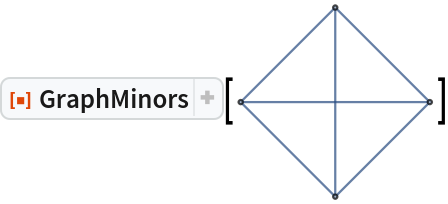Out=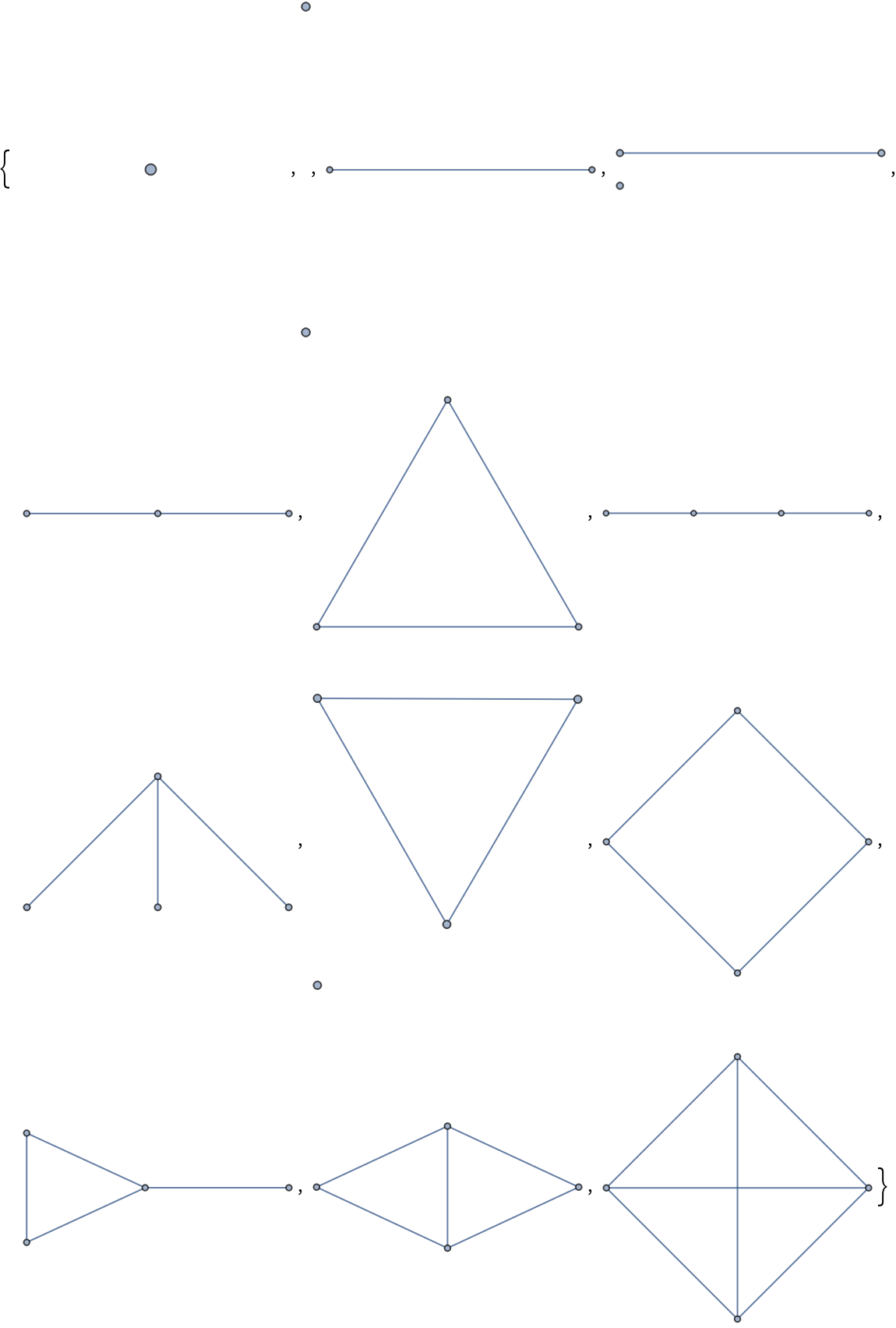### Scope (1)

Minors of the octahedral graph:

 In:=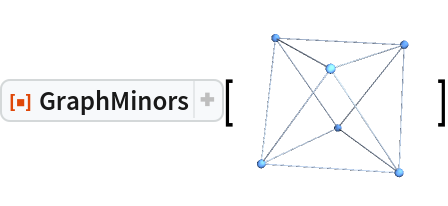Out=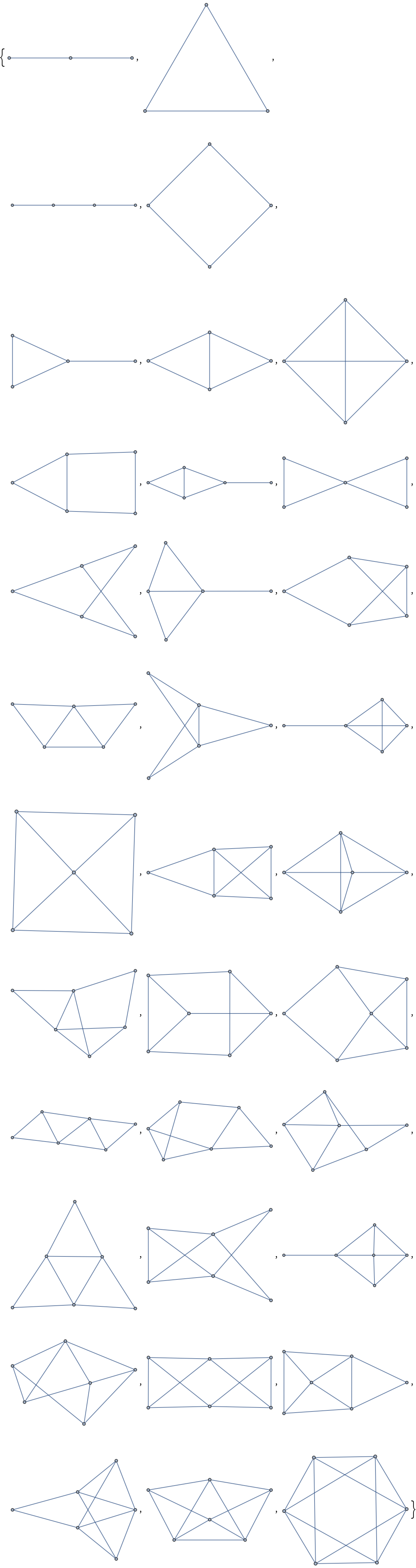### Neat Examples (2)

Demonstrate the Kuratowski reduction theorem on the 16-cell graph:

 In:=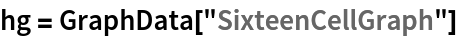Out=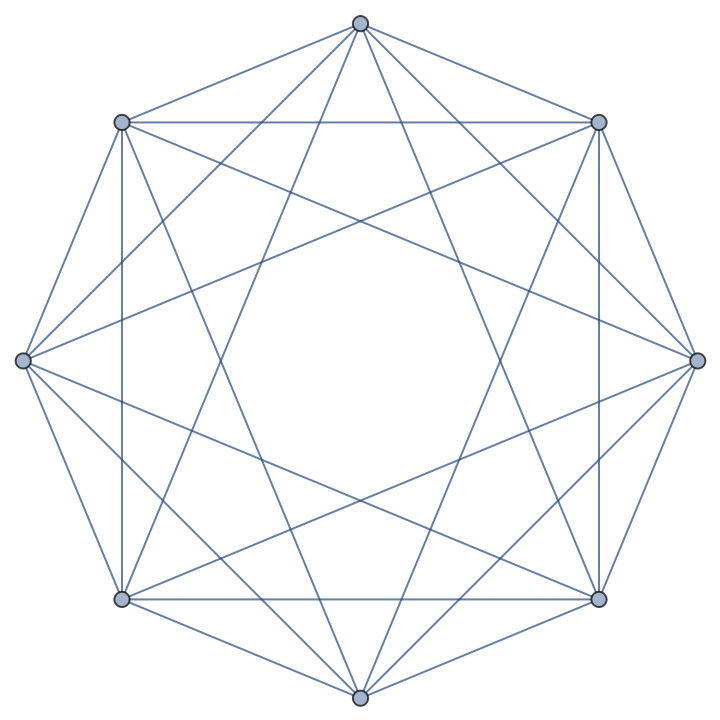In:=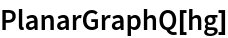Out=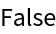The pentatope graph is one of the graph minors:

 In:=Out=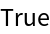## Version History

• 1.0.0 – 18 March 2020